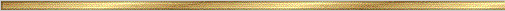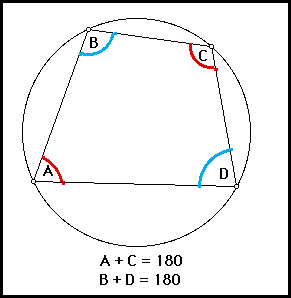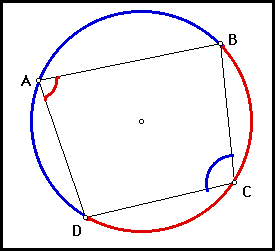### Explanation:

An inscribed quadrilateral is any four sided figure whose vertices all lie on a circle. (The sides are therefore chords in the circle!) This conjecture give a relation between the opposite angles of such a quadrilateral. It says that these opposite angles are in fact supplements for each other. In other words, the sum of their measures is 180 degrees.

For the quadrilateral shown above, this conjecture says that:

AngleA + AngleC = 180
AngleB + AngleD = 180

### The precise statement of the conjecture is:

Conjecture (Quadrilateral Sum ): Opposite angles in any quadrilateral inscribed in a circle are supplements of each other. (Their measures add up to 180 degrees.)

Proof: We use ideas from the Inscribed Angles Conjecture to see why this conjecture is true. The main result we need is that an inscribed angle has half the measure of the intercepted arc. Here, the intercepted arc for Angle(A) is the red Arc(BCD) and for Angle(C) is the blue Arc(DAB).Arc(BCD)=2*Angle(A) and Arc(DAB)=2*Angle(C)

Arc(BCD)+Arc(DAB) = 360

2*Angle(A)+2*Angle(C) = 2*[Angle(A)+Angle(C)] = 360

Angle(A)+Angle(C) = 180

The proof is complete!